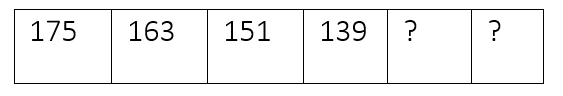Mathematics
Easy

Question

# A subtraction rule was used to make the pattern of numbers in the table.If the pattern continues, then the next two numbers will be

## 127, 116    127, 115    128, 115    129, 117Hint:

## The correct answer is: 127, 115

### The given sequence is 175, 163, 151, 139We have to find the next two terms in this sequence.Let’s find the difference between the first and second term. It will be the constant that is subtracted from all the terms.Constant = first term – second term= 175 - 163= 12So, the difference between each term is 12Let’s see how it works for every term.163 – 12 = 151151 – 12 = 139Now, let’s find the next terms.The last term from the above sequence is 139So, we will subtract 4 from the term139 – 12 = 127This will be our new first term. We will take this term and subtract 12 to get next term127 – 12 = 115We got the new terms, “127”and “115”. We will see which option matches these values.The complete sequence will be 175, 163, 151, 139, 127, 115And the option which is '127, 115’, is the required option.

For such questions, we should know the basic operations. When the rule used to write the pattern is not mentioned, we have to use all four operations to check the pattern. The operations are addition, subtraction, division, and multiplication.

### Related Questions to study#### With Turito Foundation.#### Get an Expert Advice From Turito.